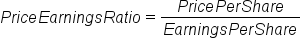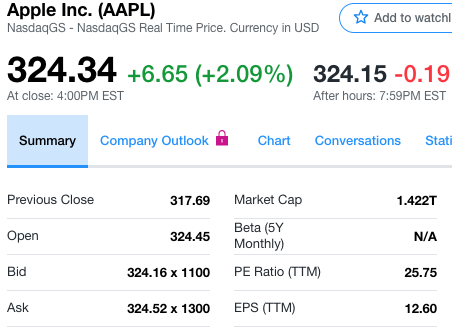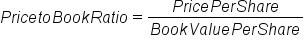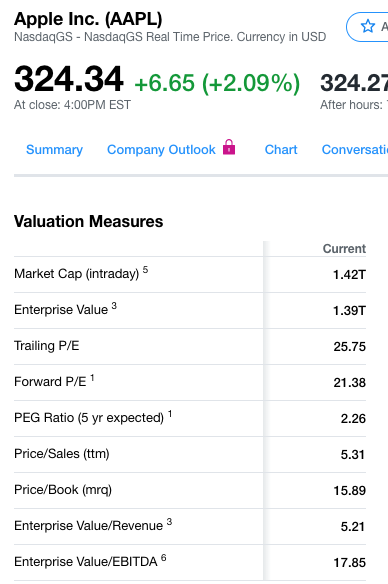+

# Market Value Ratios

Author: Sophia Tutorial
##### Description:

Interpret the ratios that measure the relative value of organizations.

(more)### Developing Effective Teams

*No strings attached. This college course is 100% free and is worth 1 semester credit.

37 Sophia partners guarantee credit transfer.

299 Institutions have accepted or given pre-approval for credit transfer.

* The American Council on Education's College Credit Recommendation Service (ACE Credit®) has evaluated and recommended college credit for 32 of Sophia’s online courses. Many different colleges and universities consider ACE CREDIT recommendations in determining the applicability to their course and degree programs.

Tutorial

what's covered
In this lesson, you will learn about ratios that measure the relative value of organizations. Specifically, this lesson will cover:
1. Price Earnings Ratio
2. Price to Book Ratio

## 1. Price Earnings Ratio

In the stock market, investors and analysts both need a way to measure the relative value of organizations. They do this by utilizing a couple of ratios:

• Price earnings ratio
• Price to book ratio
In stock trading, the price earnings ratio, also known as the PE ratio or simply the "multiple," is the measure of the market price of a company's share relative to its annual earnings per share.

The PE ratio is calculated by dividing the market price per share by the annual earnings per share.

formula

Price Earnings RatioThis ratio is a widely used valuation multiple used as a guide to the relative values of companies. If a PE ratio is higher, it means that investors are willing to pay more for each unit of current net income, so the stock is more expensive than one with a lower PE ratio.

### IN CONTEXT

Here is a screen clipping of a quote from Yahoo Finance for Apple Computer.Notice it is selling for \$324.34 a share, which is rather pricey. However, we can see that the price earnings ratio, or the multiple, is 25.75. Just underneath that, we see the earnings per share is \$12.60 annually. If we take that price of \$324.34 divided by the earnings per share of \$12.60, we get the PE ratio, the multiple, of 25.75.

So, if we are investing in tech stocks, we can compare that multiple to other companies in the industry.
term to know

Price Earnings Ratio
A ratio that measures a company’s current share price relative to its per-share earnings.

## 2. Price to Book Ratio

The second ratio that is used to capture market value is the price to book ratio. This ratio is used to compare the company's current market price to its book value.

The price to book ratio is calculated by taking the share price and dividing by the book value per share.

formula

Price to Book RatioThis ratio can vary a lot by industry. Industries that require more infrastructure capital will usually trade at price to book ratios that are lower than consulting firms or firms in the service industry. This ratio is most commonly used to compare banks, because most assets and liabilities of banks are usually valued at market value.

A higher price to book ratio implies that investors expect management to create more value from their assets. All other things considered equal, price to book ratios do not directly provide any information on the ability of the firm to generate profits or cash for shareholders. However, it does provide some idea of whether an investor is paying too much for what would be left if the company went bankrupt immediately.

### IN CONTEXT

Here again is Apple Computer and some information from Yahoo Finance.Here we can calculate a price to book ratio of 15.89 by dividing the price of \$324.34 by the book value of the firm's total capitalization. The book value per share is not shown here but we do see the total market capitalization at \$1.42 trillion. This projects the relative value of the firm.
term to know

Price to Book Ratio
A ratio that compares a company’s current market price to its book value.
summary
In this lesson, you learned that the price earnings ratio, also called the PE ratio or the "multiple," is the market price per share divided by the annual earnings per share. It is a widely used ratio in valuation of multiple organizations and is used as a guide for the relative values of companies. As an example, a higher PE ratio means investors are paying more for each unit of current income, so the stock is more expensive than one with a lower PE ratio.

The second ratio is the price to book ratio. It is calculated by dividing the company's current share price by the book value per share. A higher price to book ratio implies that investors expect management to create more value from the given set of assets, all other things being equal.

Best of luck in your learning!

Source: THIS CONTENT HAS BEEN ADAPTED FROM LUMEN LEARNING'S “MARKET VALUE RATIOS” TUTORIAL.

Terms to Know
Price Earnings Ratio

A ratio that measures a company’s current share price relative to its per-share earnings.

Price to Book Ratio

A ratio that compares a company’s current market price to its book value.

Formulas to Know
Price Earnings RatioPrice to Book RatioRating